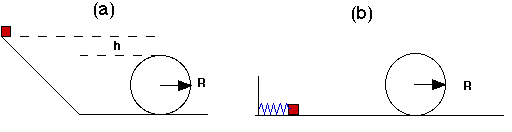# Physics problem: energy conservation around loop

• hollyrenee

#### hollyrenee

I've been fooling with this problem for quite some time now and I'm not sure where I'm going wrong. Any help would be appreciated.

## Homework Statement

The two problems below are related to a cart of mass M = 500 kg going around a circular loop-the-loop of radius R = 12 m, as shown in the figures. Assume that friction can be ignored. Also assume that, in order for the cart to negotiate the loop safely, the normal force exerted by the track on the cart at the top of the loop must be at least equal to 0.5 times the weight of the cart. (Note: This is different from the conditions needed to "just negotiate" the loop.) You may treat the cart as a point particle.a) For this part, the cart slides down a frictionless track before encountering the loop. What is the minimum height h above the top of the loop that the cart can be released from rest in order that it safely negotiate the loop?

## Homework Equations

fcp = macp = (mv2) / r
K = (1/2)mv2
(1/2)mv2 = mgh

## The Attempt at a Solution

If the cart is to go around safely, Fn is greater than or equal to .5 mg. Fcp is equal to the total forces acting on the cart at the top of the loop (I think this is where I'm going wrong...). The problem says that the normal force is (.5)500 = 250.

So I set fcp = 250 (again...I have a feeling this is wrong), so
250 = (mv2)/r = 500 kg * v2 / 12
v = 2.44 m/s

I then tried plugging this into the equation mv2 = 2mgh (which I used because the energy will be the same on both sides) and got:

500 kg * 6 m/s = 2 (500 kg) * 9.81 m/s2 * h
h = .305 m

And this is obviously the wrong answer, which is why I'm here. =]

Welcome to PF!

Hi hollyrenee! Welcome to PF!You're not applying Newton's second law correctly …

mv2/r = mass times acceleration = total force …

what is total force?Well it would be sum of forces acting on the cart, which I think is just gravity and normal force. So would gravity be positive in this case and the normal negative, since it's at the top of the loop? I already see one place that I went wrong, when I said that the normal force is at least .5(500 kg). It should be .5(500 kg * -9.81 m/s2) = -2452. N, which I think is the normal force. And then I would think the force from gravity is 4905 N. I'm a little confused about the positive and negative signs though. Sorry if this sounds really stupid, I'm a complete noob at physics. =/

Well it would be sum of forces acting on the cart, which I think is just gravity and normal force. So would gravity be positive in this case and the normal negative, since it's at the top of the loop?

I'm a little confused about the positive and negative signs though.

Hi hollyrenee!Look at it this way …

the cart is under the track at the top of the loop …

so which way does the force of gravity act?

which way does the normal force act?Well I ended up getting the answer, but still don't understand if the forces are supposed to be positive or negative. I just set the Fn and Fg both as positive (because v2 must be positive if SF = (mv2) / r), added them together, and plugged them into the equations solving for h.

So since the track is above the cart, I was thinking that the normal force would act downward, as well as the force from gravity. Is that why I was just able to set them both as positive? Or are they originally just positive? Or am I just over-thinking this and confusing myself? =/

And thank you for the help btw!

So since the track is above the cart, I was thinking that the normal force would act downward, as well as the force from gravity. Is that why I was just able to set them both as positive? Or are they originally just positive? Or am I just over-thinking this and confusing myself? =/

Hi hollyrenee!Yes, they both act in the same direction, so you add them.

I suspect you're getting confused by the word "positive", especially since both forces act downwardsRemember, it doesn't matter which direction you choose to be "positive", so long as you stick to the same choice for everything.Aghh, yes the word positive threw me off. :grumpy: But I guess there's really no such thing as "positive" unless you're relating it to another direction. (at least as far as introductory physics...maybe some far advanced theory has developed an actual positive direction?). Thanks again!